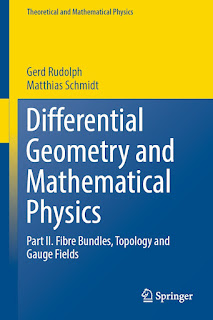GEOMETRY MATHEMATICS

# Differential Geometry and Mathematical Physics Part II Fibre Bundles, Topology and Gauge Fields PDF Free DownloadThe book is devoted to the study of the geometrical and topological structure of gauge theories. It consists of the following three building blocks:- Geometry and topology of fibre bundles,- Clifford algebras, spin structures and Dirac operators,- Gauge theory. Written in the style of a mathematical textbook, it combines a comprehensive presentation of the mathematical foundations with a discussion of a variety of advanced topics in gauge theory. The first building block includes a number of specific topics, like invariant connections, universal connections, H-structures and the Postnikov approximation of classifying spaces. Given the great importance of Dirac operators in gauge theory, a complete proof of the Atiyah-Singer Index Theorem is presented.